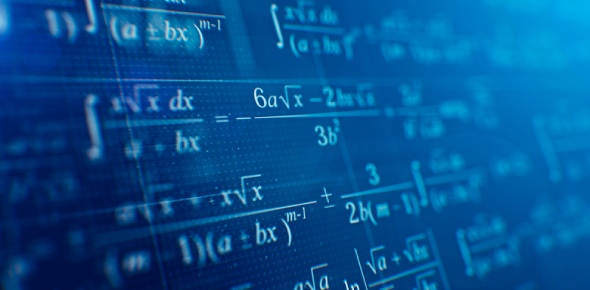# Mathematician Test

7 Questions | Total Attempts: 1902SettingsRelated Topics
• 1.
Which mathematician is considered the Father of Geometry?
• A.

Brahmagupta

• B.

Euler

• C.

Euclid

• D.

Newton

• 2.
Archimedes is credited with coining which of the following phrases?
• A.

"Eureka!"

• B.

"I wanna go fast!"

• C.

"A penny saved is a penny earned."

• D.

"I love math!"

• 3.
Hypatia is the first first female mathematician of record.
• A.

True

• B.

False

• 4.
Besides Newton, which of the following mathematicians is also credited with discovering Calculus?
• A.

Leibniz

• B.

Descartes

• C.

Euclid

• D.

Euler

• 5.
Euler made significant findings in the realm of number theory which includes prime and perfect numbers.  Which one of the following is a perfect number?
• A.

28

• B.

25

• C.

101

• D.

7

• 6.
Brahmagupta is credited with naming zero.
• A.

True

• B.

False

• 7.
Which of the following mathematicians is credited with inventing the Cartesian coordinate system (the xy-plane)?
• A.

Descartes

• B.

Newton

• C.

Leibniz

• D.

Euler